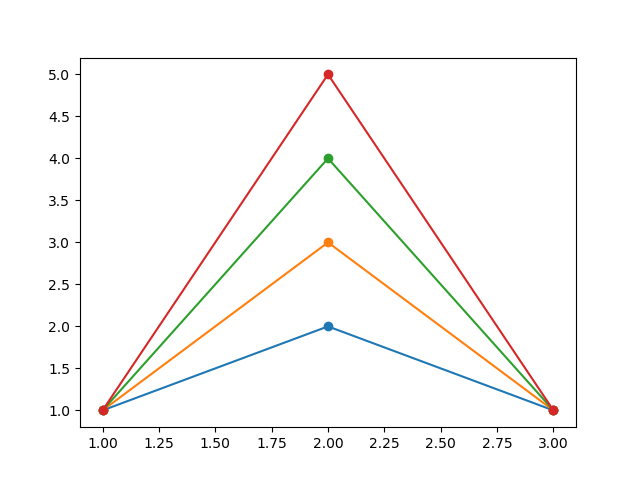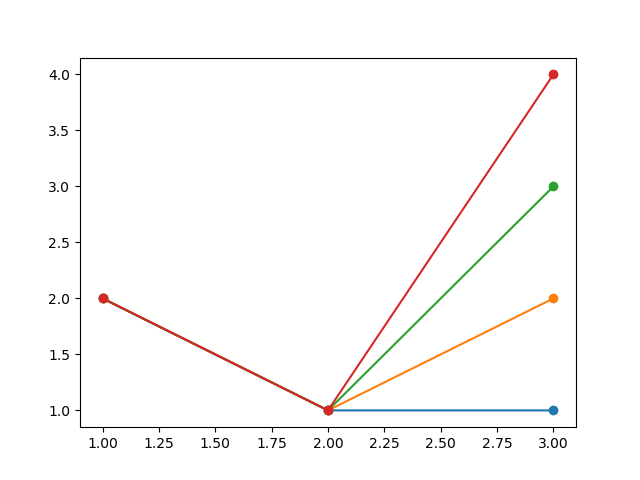# Interactive Fortran Compiler¶

## Calculator¶

In :
1+3

Out:
4
In :
(2+3)*3

Out:
15

## Global Variables¶

In :
integer :: i

In :
integer :: j

In :
i = 5
j = 6

In :
i

Out:
5
In :
(i+j)*3

Out:
33

## Functions¶

You can declare a function and use it in expressions:

In :
integer function fn(a, b)
integer, intent(in) :: a, b
fn = a + b
end function

In :
fn(2, 3)

Out:
5
In :
fn(2, 3)*3

Out:
15

You can redeclare a function and it will shadow the old fn function. Now fn(2, 3) returns a different value:

In :
integer function fn(a, b)
integer, intent(in) :: a, b
fn = a - b
end function

fn(2, 3)

Out:
-1

## Control Flow, Printing¶

You can use loops, if statements or the print function.

In :
integer :: i
do i = 1, 4
if (i == 3) cycle
print *, "variable i =", i
end do

variable i = 1
variable i = 2
variable i = 4


# Plotting¶

You can do plotting using Matplotlib underneath:

In :
integer :: i, tmp
do i = 1, 4
tmp = plot(1, i+1, 1)
end do
show()# Magic Commands¶

### Compiler¶

In :
integer :: i, j, n
n = 5
j = 0


Show Abstract Syntax Tree (AST) after parsing (based on syntax only, no semantics):

In :
%%showast
do i = 1, n
j = j + i
end do

Parse AST:
DoLoop(head=do_loop_head(var='i', start=Num(n='1'), end=Name(id='n'), increment=None), body=[Assignment(target=Name(id='j'), value=BinOp(left=Name(id='j'), op=Add(), right=Name(id='i')))])

Show LLVM code:

In :
%%showllvm
do i = 1, n
j = j + i
end do

LLVM IR (optimized):
; ModuleID = '<string>'
source_filename = "<string>"
target triple = "unknown-unknown-unknown"

@i_0 = external local_unnamed_addr global i64
@j_0 = external local_unnamed_addr global i64
@n_0 = external local_unnamed_addr global i64

; Function Attrs: norecurse nounwind
define i64 @_run16() local_unnamed_addr #0 {
.entry:
store i64 0, i64* @i_0, align 4
%.73 = load i64, i64* @n_0, align 4
%.84 = icmp slt i64 %.73, 1
br i1 %.84, label %loop.end, label %loop.body.lr.ph

loop.body.lr.ph:                                  ; preds = %.entry
%j_0.promoted = load i64, i64* @j_0, align 4
br label %loop.body

loop.body:                                        ; preds = %loop.body.lr.ph, %loop.body
%.156 = phi i64 [ %j_0.promoted, %loop.body.lr.ph ], [ %.15, %loop.body ]
%.65 = phi i64 [ 1, %loop.body.lr.ph ], [ %.6, %loop.body ]
%.15 = add i64 %.156, %.65
%.6 = add i64 %.65, 1
%.8 = icmp sgt i64 %.6, %.73
br i1 %.8, label %loop.header.loop.end_crit_edge, label %loop.body

store i64 %.65, i64* @i_0, align 4
store i64 %.15, i64* @j_0, align 4
br label %loop.end

loop.end:                                         ; preds = %loop.header.loop.end_crit_edge, %.entry
ret i64 undef
}

attributes #0 = { norecurse nounwind }


Show assembly code:

In :
%%showasm
do i = 1, n
j = j + i
end do

ASM:
.text
.file	"<string>"
.globl	_run17                  # -- Begin function _run17
.p2align	4, 0x90
.type	_run17,@function
_run17:                                 # @_run17
# %bb.0:                                # %.entry
movabsq	$i_0, %rax movq$0, (%rax)
movabsq	$n_0, %rcx movq (%rcx), %rcx testq %rcx, %rcx jle .LBB0_4 # %bb.1: # %loop.body.lr.ph movabsq$j_0, %rdx
movq	(%rdx), %rsi
movl	\$1, %edi
.p2align	4, 0x90
.LBB0_2:                                # %loop.body
# =>This Inner Loop Header: Depth=1
incq	%rdi
cmpq	%rcx, %rdi
jle	.LBB0_2
decq	%rdi
movq	%rdi, (%rax)
movq	%rsi, (%rdx)
.LBB0_4:                                # %loop.end
retq
.Lfunc_end0:
.size	_run17, .Lfunc_end0-_run17
# -- End function

.section	".note.GNU-stack","",@progbits


### Filesystem¶

Load and show an image file:

In :
integer :: i, tmp
do i = 1, 4
tmp = plot(2, 1, i)
end do
savefig(1)

Saving to output1.png.

Out:
0
In :
%showimage output1.pngList the current directory:

In :
%ls

total 84
-rw-r--r-- 1 certik certik  5774 Nov 16 14:21 Demo.ipynb
-rw-r--r-- 1 certik certik 27981 Nov 29 12:49 output1.png
-rw-r--r-- 1 certik certik 45214 Nov 29 12:49 show_output1.png